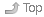# 1 Final Exam Schedule PostedIndex• The Final Exam date is posted on the Course Home Page.
• For full mark credit, read Test Instructions for important directions on how to enter your answers on the mark-sense forms.

# 2 Lecture Notes for This WeekIndex## 2.2 From Blackboard Course DocumentsIndexThese documents have restricted distribution and cannot be put on the Course Home Page.

• 01.ppt - Introduction
• ignore the history sections
• vonNeumann architectures: the vonNeumann bottleneck
• CPU components
• 02.ppt - Data Representation
• CPU Flags: slides 47-49, 53-54
• slide 54 has an error - change second-line 0100+0010 to be 0100+0110
• 03.ppt - Boolean Algebra and Digital Logic
• Ignore: Digital Logic

## 2.4 From the Classroom Whiteboard/ChalkboardIndex• Your in-class notes go here.

• What you might need is a Binary Watch - see image.Binary Watch (photo courtesy of Lucas)

• how many ASCII characters are there?
• what is the difference between ‘A’ and ‘a’? ‘M’ and ‘m’? ‘Z’ and ‘z’?
• how many ASCII control characters (unprintable) are there?
• what are their hex values?
• bitwise operators: `AND OR XOR NOT` -> `& | ^ ~`
• a=6,b=3 what is `a&b`? what is `a|b`? what is `a^b`? what is `~a`?
• a=5,b=10 what is `a&b`? what is `a|b`? what is `a^b`? what is `~a`?
• ASCII parity bit, used to detect errors in data transmission
• is ‘A’ even or odd parity? convert it to even parity, then to odd
• is CR even or odd parity? convert it to even parity, then to odd
• vonNeumann architecture limitations (from 01.ppt)
• what is the vonNeumann bottleneck?
• basic CPU components (from 01.ppt)
• Carry and Overflow flags (continued)
• see the Class Notes, above, and the 02.ppt slides, above
• The Overflow flag comes on when the answer is wrong for two’s complement (signed). The simple rule to remember is that Overflow flag only turns on when pos+pos=neg or neg+neg=pos.
• For subtraction, note that subtracting a positive is the same math as adding a negative, and subtracting a negative is the same math as adding a positive:
• neg-pos = neg+neg and Result must also be negative (else Overflow)
• pos-neg = pos+pos and Result must also be positive (else Overflow)
• Adding two negatives must give a negative; adding two positives must give a positive. Subtracting a positive from a negative must generate a more negative number, not a positive number, and subtracting a negative value from a positive number must give a more positive number, not a negative number. (Otherwise: Overflow)
• The Carry flag indicates a Borrow when doing subtraction of a big number from a smaller number, e.g. 2-3
• The Little-Man Computer
``````Author:
| Ian! D. Allen  -  idallen@idallen.ca  -  Ottawa, Ontario, Canada